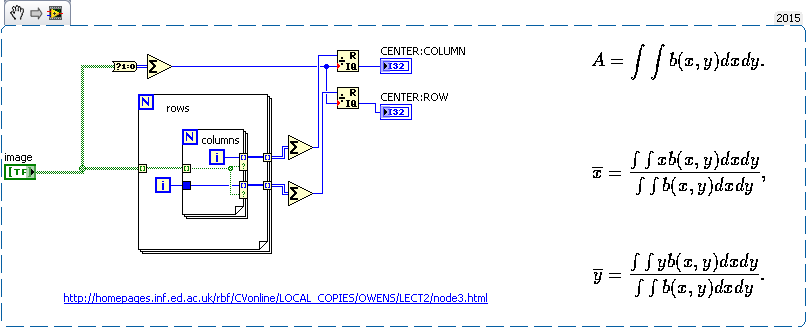# LabVIEW

cancel
Showing results for
Did you mean:

## Fit rectangle into Binary Particle

Hello all,

I have a problem with a function I am trying to implement: I would like to get the biggest Rectangle that fits into a region in my image. By biggest I mean the biggest area. Here's a graphic to illustrate:My form will look something like the black one (inmy Image the entire form is filled with white) and I would like my result to look something like the green rectangle.

So far I have tried the following: Detect falling edge from top to bottom gives me the lower line. I then rotate the image so the lower line is horizontal to avoid using math. Then I use the base line to detect intersects with the side from where I draw up to get the upper points. Then I select the correct point and draw the rectangle.

This works fine for some images, but others are a rotated or a corner might be missing which is when it gives me bad results.

Does anyone have a pointer as to how to better implement this? Any help is greatly appreciated!

Remember Cunningham's Law
Message 1 of 9
(2,240 Views)

## Re: Fit rectangle into Binary Particle

Do you need just the size/area, or you need the coordinates too?Message 2 of 9
(2,206 Views)

## Re: Fit rectangle into Binary Particle

Hi Stockson,

that really depends on the difficulty. In general the size with width and height would be enough. I would also like to visualize the rectangle, but that's more a nice to have.

Remember Cunningham's Law
Message 3 of 9
(2,192 Views)

## Re: Fit rectangle into Binary Particle

So, you are looking for the maximum rectangle within the black border?

How fast does your solution need to be?

Message 4 of 9
(2,186 Views)

## Re: Fit rectangle into Binary Particle

Yes, I'm looking for the rectangle with the biggest area within the black shape (in my sketch).

The solution does not have to be very fast. If it completes within a couple of seconds it's OK.

Remember Cunningham's Law
Message 5 of 9
(2,172 Views)

## Re: Fit rectangle into Binary Particle

Could you provide some real data?

e.g. is the object always  symmetric?

Message 6 of 9
(2,155 Views)

## Re: Fit rectangle into Binary Particle

So here's my suggestion:

I would try to calculate the two eigenvectors of the binary image, taking only the object-pixels in consideration.

This will give you a direction and a length for each of the two image-eigenvectors.

Furthermore, it might be helpful to calculate the center of mass, which is the sum of the objects row indices diveded by the number of object pixels and the sum of the objects column indices  diveded by the number of object pixels

I would use this Information as a starting point of finding the maximum rectangle within the object.

Message 7 of 9
(2,130 Views)

## Re: Fit rectangle into Binary Particle

Hi Alex,

thanks for the suggestion.

To be totally honest I didn't get much of that. What use will the eigenvectors be towards finding the rectangle? And how would I calculate them?

Because I understand so little of that it seems a quicker solution to just refine my other algorithm, so it works even for heavily distorted images.

As for the center of mass, I can just get that from the particle anylsis, can't I?

Remember Cunningham's Law
Message 8 of 9
(2,115 Views)

## Re: Fit rectangle into Binary Particle

@PeterFoerster wrote:

Hi Alex,

thanks for the suggestion.

To be totally honest I didn't get much of that. And how would I calculate them?

Because I understand so little of that it seems a quicker solution to just refine my other algorithm, so it works even for heavily distorted images.

As for the center of mass, I can just get that from the particle anylsis, can't I?

What use will the eigenvectors be towards finding the rectangle?

I thought, maybe there is a connection between the length of the eigenvector and the length of the rectangle you are looking for.

It's a guess, I might be completly wrong...

If one of the rectangle's sides -we are looking for- is parallel to the axis of rotation, the greater one of the two eigenvector will be parallel towards this axis of rotation.

And how would I calculate them?

It can be done using the built-in LabView function

http://zone.ni.com/reference/en-XX/help/371361J-01/gmath/eigenvalues_and_vectors/

...in combination with:

http://zone.ni.com/reference/en-XX/help/371361J-01/gmath/covariance_matrix/

and:

http://zone.ni.com/reference/en-XX/help/371361H-01/gmath/a_x_b/

here's another approach: http://homepages.inf.ed.ac.uk/rbf/CVonline/LOCAL_COPIES/OWENS/LECT2/node3.html

As for the center of mass, I can just get that from the particle anylsis, can't I?

I don't know if the particle analysis is capable of doing this, but in general, calculating the (optical) center of mass,

is quite straight forward:Message 9 of 9
(2,100 Views)